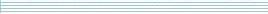| home      | people     | research     | publications    | seminars    | events     | contactCommunication Networks   | Systems Biology   | Hybrid Systems    | Machine Learning   | Dynamics & Interaction### Schools Mathematics Grand Challenge

Week nine's Puzzles

Problem 19:

The nineteenth problem was:

John was born in the 20th century. On one of John's birthdays the age he turned was equal to the sum of the 4 digits in the year of that birthday and also equal to the sum of the 4 digits in the year he was born. What age did John turn on this birthday?

The solution was:

John was born in the 20th century, so a year like 19ab. In some year John had a birthday so that his age was (1 + 9 + a + b). This was also the sum of the digits of the year of his birthday.

First, since 1 + 9 + a + b is at most as big as 1 + 9 + 9 + 9, we know John must have been no more than 28 years old when this happened. We also know 1 + 9 + a + b is at least 1 + 9 + 0 + 0, so John was at least 10.

In problems with sums of digits, it is sometimes a good to think about the remainder when you divide by 9. This is because if you sum the digits of a number the answer will have the same remainder when you divide by 9. For example:

87253 / 9 = 9694 remainder 7

(8+7+2+5+3) / 9 = 25 / 9 = 2 remainder 7

We know the year John was born has the same sum of digits as the year of John's birthday. This means that both these numbers have the same remainder when you divide by 9. So John's age, which is the difference of these numbers, must be divisible by 9.

We already figured out that John was between 10 and 28 years old, and so he must be either 18 or 27 years old, as these are the only ages divisible by 9 in this range.

Let's see if John could be 18. We need 1 + 9 + a + b = 18, so let's try a = 4 and b = 4. So, John would have been born in 1944 and his birthday would have been 18 years later in 1962 and 1 + 9 + 6 + 2 is 18. So, John could have been 18.

How about 27? Here we need 1 + 9 + a + b = 27, or a + b = 17. There are only two possibilities here. The first is a = 8, b = 9, John would be born in 1989 and his 27th birthday would be in 2016, but 2 + 0 + 1 + 6 = 9, and we wanted 27.

The other possibility is that a = 9 and b = 8. In this case John would have been born in 1998 and his 27th birthday would be in 2025, but again 2 + 0 + 2 + 5 = 9, and we wanted 27.

So, the only possibility is that John was 18. (Though there are many years he could have been born.)

Problem 20:

The twentieth problem was:

What remainder do you get when you divide 10^(1,000,001) - 10^(1,000,000) by 11? So we want the remainder when you divide (10 to the power of 1,000,001) minus (10 to the power of 1,000,000) by 11. Your answer should be a whole number between 0 and 10.

HINT: An old tip of the week might be useful.

The solution was:

Do you remember this tip of the week?

If you add 1 to any odd power of 10, the number you get is divisible by 11. On the other hand, if you subtract 1 from any even power of 10, the number you get is divisible by 11.

It tells us that 10^(1,000,001) + 1 is divisible by 11, and tells us that 10^(1,000,000) - 1 is divisible by 11. This means that

(10^(1,000,001) + 1) - (10^(1,000,000) - 1)

is divisible by 11. We can rearrange this a little to get

10^(1,000,001) - 10^(1,000,000) + 2

is divisible by 11. That means 10^(1,000,001) - 10^(1,000,000) is two short of being divisible by 11, and so has remainder 9.